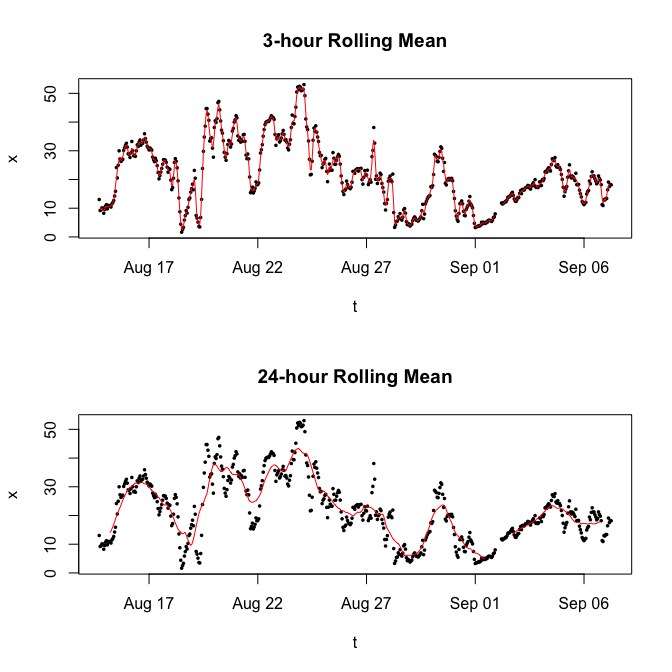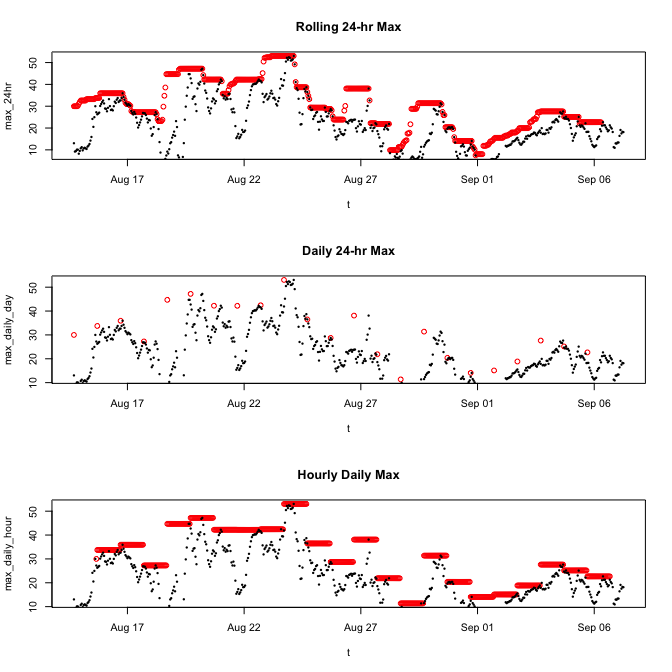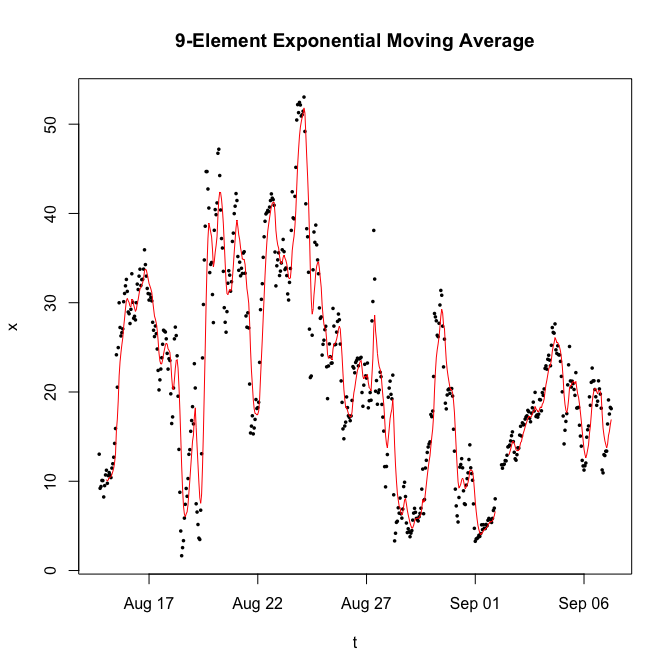# Introduction to MazamaRollUtils

## Background

Analysis of time series data often involves applying “rolling” functions to calculate, e.g. a “moving average”. These functions are straightforward to write in any language and it makes sense to have C++ versions of common rolling functions available to R as they dramatically speed up calculations. Several packages exist that provide some version of this functionality:

• zoo – core R package with a specific data model
• seismicRoll – rolling functions focused on seismology
• RcppRoll – rolling functions for basic statistics

Our goal in creating a new package of C++ rolling functions is to build up a suite of functions useful in environmental time series analysis. We want these functions to be available in a neutral environment with no underlying data model. The functions are as straightforward to use as is reasonably possible with a target audience of data analysts at any level of R expertise.

## Installation

Install from CRAN with:

install.packages('MazamaRollUtils')

devtools::install_github("MazamaScience/MazamaRollUtils")

## Features

### Predictable Names

Many of the rolling functions in MazamaRollUtils have the names of familiar R functions with roll_ prepended. These functions calculate rolling versions of the expected statistic:

• roll_max()
• roll_mean()
• roll_median()
• roll_min()
• roll_prod()
• roll_sd()
• roll_sum()
• roll_var()

Additional rolling functions with no equivalent in base R include:

• roll_MAD() – Median Absolute Deviation
• roll_hampel() – Hampel filter

Other functions wrap the rolling functions to provide enhanced functionality. These are not required to return vectors of the same length as the input data.

• findOutliers() – returns indices of outlier values identified by roll_hampel().

### Common Arguments

All of the roll_~() functions accept the same arguments where appropriate:

• x – Numeric vector input.
• width – Integer width of the rolling window.
• by – Integer shift to use when sliding the window to the next location align Character position of the return value within the window. One of: "left" | "center" | "right".
• na.rm – Logical specifying whether values should be removed before the calculations within each window.

The roll_mean() function also accepts:

• weights – Numeric vector of size width specifying each window index weight. If NULL, unit weights are used.

### Predictable Return Length

The output of each roll_~() function is guaranteed to have the same length as the input vector, with varying stretches of NA at one or both ends depending on arguments width, align and na.rm. This makes it easy to align the return values with the input data.

## Examples

The example dataset included in the package contains a tiny amount of data but suffices to demonstrate usage of package functions.

### Basic Rolling Means

library(MazamaRollUtils)

# Extract vectors from our example dataset
t <- example_pm25$datetime x <- example_pm25$pm25

# Plot with 3- and 24-hr rolling means
layout(matrix(seq(2)))
plot(t, x, pch = 16, cex = 0.5)
lines(t, roll_mean(x, width = 3), col = 'red')
title("3-hour Rolling Mean")
plot(t, x, pch = 16, cex = 0.5)
lines(t, roll_mean(x, width = 24), col = 'red')
title("24-hour Rolling Mean")layout(1)

### Using ‘width’, ‘align’, ‘by’ and ‘na.rm’

The next example uses all of the standard arguments to quickly calculate a daily maximum value and spread it out across all indices.

library(MazamaRollUtils)

# Extract vectors from our example dataset
t <- example_pm25$datetime x <- example_pm25$pm25

# Calculate the left-aligned 24-hr max every hour, ignoring NA values
max_24hr <- roll_max(x, width = 24, align = "left", by = 1, na.rm = TRUE)

# Calculate the left-aligned daily max once every 24 hours, ignoring NA values
max_daily_day <- roll_max(x, width = 24, align = "left", by = 24, na.rm = TRUE)

# Spread the max_daily_day value out to every hour with a right-aligned look "back"
max_daily_hour <- roll_max(max_daily_day, width = 24, align = "right", by = 1, na.rm = TRUE)

# Plot with 3- and 24-hr rolling means
layout(matrix(seq(3)))
plot(t, max_24hr, col = 'red')
points(t, x, pch = 16, cex = 0.5)
title("Rolling 24-hr Max")
plot(t, max_daily_day, col = 'red')
points(t, x, pch = 16, cex = 0.5)
title("Daily 24-hr Max")
plot(t, max_daily_hour, col = 'red')
points(t, x, pch = 16, cex = 0.5)
title("Hourly Daily Max")layout(1)

### Using roll_mean() with ‘weights’

The roll_mean() function accepts a weights argument that can be used to create a weighted moving average. The next example demonstrates creation of an exponential weighting function to be applied to our data.

library(MazamaRollUtils)

# Extract vectors from our example dataset
t <- example_pm25$datetime x <- example_pm25$pm25

# Create weights for a 9-element exponentially weighted window
#   See:  https://en.wikipedia.org/wiki/Moving_average
N <- 9
alpha <- 2/(N + 1)
w <- (1-alpha)^(0:(N-1))
weights <- rev(w)          # right aligned window

EMA <- roll_mean(x, width = N, align = "right", weights = weights)

# Plot Exponential Moving Average (EMA)
plot(t, x, pch = 16, cex = 0.5)
lines(t, EMA, col = 'red')
title("9-Element Exponential Moving Average")